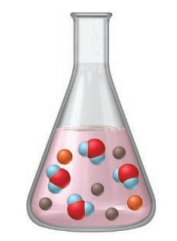# Problem: The flask represents the products of the titration of 25 mL of sulfuric acid with 25 mL of sodium hydroxide.If each orange sphere represents 0.010 mol of sulfate ion, how many moles of acid and of base reacted?

###### FREE Expert Solution

We are asked how many moles of acid and of base reacted?

sulfuric acid → H2SO4

sodium hydroxide → NaOH

Reaction:

2 NaOH +  H2SO4 → NaSO4 + 2 H2O

2 orange spheres →  0.020 mol SO42-

99% (236 ratings)###### Problem Details

The flask represents the products of the titration of 25 mL of sulfuric acid with 25 mL of sodium hydroxide.If each orange sphere represents 0.010 mol of sulfate ion, how many moles of acid and of base reacted?